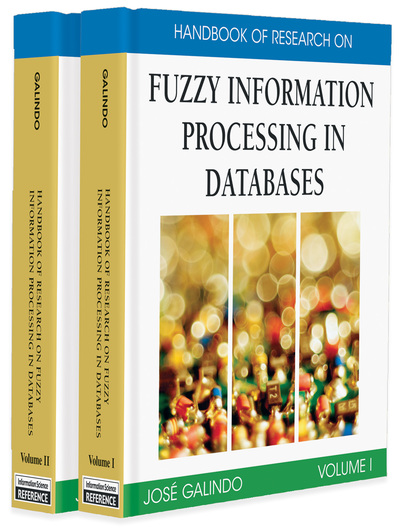# Data Dependencies in Codd's Relational Model with Similarities

Radim Belohlavek (Binghamton University–SUNY, USA) and Vilem Vychodil (Palacky University, Czech Republic)
DOI: 10.4018/978-1-59904-853-6.ch025
Available
\$37.50
No Current Special Offers

## Abstract

This chapter deals with data dependencies in Codd’s relational model of data. In particular, we deal with fuzzy logic extensions of the relational model that consist of adding similarity relations to domains and consider functional dependencies in these extensions. We present a particular extension and functional dependencies in this extension that follow the principles of fuzzy logic in a narrow sense. We present selected features and results regarding this extension. Then, we use this extension as a reference model and compare it to several other extensions proposed in the literature. We argue that following the principles of fuzzy logic in a narrow sense, the same way we can follow the principles of classical logic in the case of the ordinary Codd relational model, helps achieve transparency, versatility, conceptual clarity, and theoretical and computational tractability of the extension. We outline several topics for future research.

## Key Terms in this Chapter

Armstrong Rules: Armstrong rules are deduction rules for reasoning with functional dependencies. Usually, by Armstrong rules we mean a collection of rules that are syntactico-semantically complete. That is, a functional dependency semantically follows from a set T of functional dependencies iff can be obtained from T using Armstrong rules.

Functional dependency: Functional dependency is a formula where A and B are collections of attributes. being true in a table means that every two rows of a table that have the same values on attributes from A have the same values on attributes from B. Functional dependencies play important roles in the design of relational databases.

Structure of Truth Degrees: It is a set of truth degrees such as [0,1] equipped with truth functions of logical connectives. For instance, for the connective of implication, one can use (Lukasiewicz implication), or for and for a > b (Goguen implication). There are many choices of truth functions of logical connectives. However, the chosen collection of connectives should obey reasonable properties such as the adjointness property, which is required to be satisfied by the truth functions of conjunction and implication.

Codd’s Relational Model of Data: A theoretical model of data representation and manipulation by Edgar F. Codd (1960s, 1970s). Data are conceived as represented by tables in Codd’s model. A formal counterpart of a table is that of a relation. Data manipulation corresponds to performing operations with relations. Codd’s model relies on first-order logic and a mathematical concept of a relation. Codd’s relational model is the theoretical backbone of relational databases.

Domain with Similarity: A domain is a set of all possible values an attribute may take. For instance, a domain of the attribute age is a set {0,1,2,...,150}. A domain with similarity is a domain equipped with a particular binary fuzzy relation on it, called a similarity relation, that is, with a function assigning to every two elements of the domain a degree to which the two values are similar.

## Complete Chapter List

Search this Book:
Reset